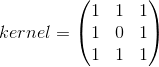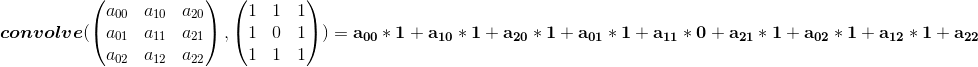# Conway's Game of Life using ArrayFire

Conway's Game of Life is a popular zero player cellular automaton devised by the John Horton Conway in 1970. The game makes for a fun evolution as the player sets the initial condition and then observes the evolution of the game.

Each cell has 2 states: live or dead. There are 4 simple rules that determine this:

• Any live cell with fewer than two live neighbours dies, as if caused by under-population.
• Any live cell with two or three live neighbours lives on to the next generation.
• Any live cell with more than three live neighbours dies, as if by overcrowding.
• Any dead cell with exactly three live neighbours becomes a live cell, as if by reproduction.

From a programmer's perspective, the game is closer to an image processing algorithm. The "world" is a 2D image, where each pixel is a cell.

The state of a cell at any single moment in time is solely determined by the number of neighbors at the previous step. Hence this makes for an embarrassingly parallel image processing algorithm. Ding ding ding! GPUs!!!

The number of neighbors can be computed using a convolution kernel:Since the value of the a cell can be represented as 1 (live) and 0 (dead), using convolution will give us the number of neighbors of any cell. The center value of the kernel is 0 as we do not want to count the cell itself.

Here is a general overview of a convolution operation:Plugging in 1s or 0s into the A matrix, we can see that this convolution operation will give us the neighborhood of each cell.

The next step is to compute the next state of the cell. Lets convert the rules into pseudocode.

```if(cell == 1 && neighbours < 2)                       -> cell = 0
if(cell == 1 && (neighbours == 2 or neighbours == 3)) -> cell = 1
if(cell == 1 && neighbours > 3)                       -> cell = 0
if(cell == 0 && neighbours == 3)                      -> cell = 1
```

But we can optimize these as below:

```if((cell == 1 || cell == 0) && neighbours < 2)  -> cell = 0 // Since this does not make a difference to dead cell
if(cell == 1 && neighbours == 2)                -> cell = 1
if((cell == 1 || cell == 0) && neighbours == 3) -> cell = 1 // Since in both cell cases, the cell is alive in the next state
if((cell == 1 || cell == 0) && neighbours > 3)  -> cell = 0 // Since in dead cell can't become live with more that 3 neighbours and an alive cell would die
```

Removing redundant conditions:

```if(neighbours < 2)                  -> cell = 0
if(cell == 1 && neighbours == 2)    -> cell = 1
if(neighbours == 3)                 -> cell = 1
if(neighbours > 3)                  -> cell = 0
```

Let's say A is the current state of the cell and neighborhood values are in matrix NHood.
We can compress the conditions into the following code:

```NHood = convolve(A, kernel);
cond0 = (NHood < 2 ) // All cells with less than 2 neighbours will have value 1, others will be 0
cond1 = (NHood == 2) // All cells with exactly 2 neighbours will have value 1, others will be 0
cond2 = (NHood == 3) // All cells with exactly 3 neighbours will have value 1, others will be 0
cond3 = (NHood > 3 ) // All cells with more than 3 neighbours will have value 1, others will be 0
```

But here is the best optimization--only two of these conditions matter: cond1 and cond2.

Using pseudocode:

```(NHood < 2 || NHood > 3) is equivalent to !(NHood == 2 && NHood == 3)
//Or
!(cond0 + cond3) = cond1 * cond2.
```

Let's see how we can compute the next state using just these two conditions.

For cond2 it is straightforward. Any cell that is 1 in cond2 will be 1 in the next state irrespective of its value in A.

For cond1, all alive cells in A with 1 in cond1 will remain 1. All cells become dead if the corresponding value in cond1 is 0. This condition can be satisfied by doing (A * cond1).
That is,

` if(A && cond2) = A, else A = 0. `

Hence the next state can be computed by:

`A = A * cond1 + cond2;`

And this is what ArrayFire is best at. Given its powerful API of element-wise operations and image processing algorithms, implementing the Game of Life is a breeze. Below is the entire ArrayFire code that is required to run Game of Life.

```// Initialize the kernel array just once
static const float h_kernel[] = {1, 1, 1,
1, 0, 1,
1, 1, 1};
static const af::array kernel(3, 3, h_kernel, af::afHost);
int wId = -1;

// Generate a random starting state
static const int game_w = 100, game_h = 100;
array state = (af::randu(game_h, game_w, f32) > 0.33).as(f32);  // Generates a 0s and 1s array based on random values
while(wId != -2) {
// Convolve gets neighbors
af::array nHood = convolve(state, kernel, false);

// Generate conditions for life
af::array C0 = (nHood == 2);
af::array C1 = (nHood == 3);

// Update state
state = state * C0 + C1;

// Display
wId = image(state, wId, "Conway Using ArrayFire");
}
```

This code is fully platform independent as it uses ArrayFire.

Let us know if you have made any cool applications using ArrayFire.

1.1.